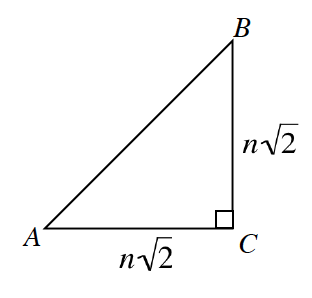### Home > PC3 > Chapter 2 > Lesson 2.2.2 > Problem2-66

2-66.Use $∆ABC$ at right to complete the following problems.

1. Calculate the exact length of $\overline {AB}$ in terms of $n$

$AB^2 = (n\sqrt{2})^2 + (n\sqrt{2})^2$

$AB^2 = 2n^2+2n^2$

1. If $n=2$, what is the value of $\sin(A)$? If $n=12$, what is the value of $\sin(A)$?

$\operatorname {sin}(A) = \frac{\text{opposite}}{\text{hypotenuse}}$

2. In terms of $n$, what is the value of $\sin(A)$?

${\frac{\sqrt{2}}{2}}$

3. What does this tell you about $\sin(45°)$

It does not matter what the side length is as long as $A=45°$.

4. What about $\cos(45°)$?

$\cos(45°)=\sin(45°)$